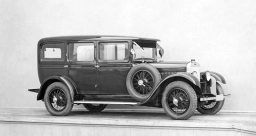# Acceleration 80626

The car stopped at an initial speed of 90 km/h on a track of 62.5 m. What was its acceleration, and how long did it take to brake the car?

a =  -5 m/s2
t =  5 s

### Step-by-step explanation:Did you find an error or inaccuracy? Feel free to write us. Thank you!

Tips for related online calculators
Need help calculating sum, simplifying, or multiplying fractions? Try our fraction calculator.
Do you want to convert velocity (speed) units?
Do you want to convert time units like minutes to seconds?